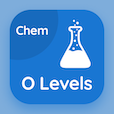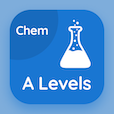Cambridge Online Courses (GCSE)

O Level Chemistry Quizzes

O Level Chemistry Quiz - Complete

Practice Basic Acidic Neutral and Amphoteric quiz questions and answers, basic acidic neutral and amphoteric MCQ questions PDF, test 138 to study O Level Chemistry online course. Acids and Bases MCQ questions, basic acidic neutral and amphoteric Multiple Choice Questions (MCQ Quiz) for online college degrees. Basic Acidic Neutral and Amphoteric Book PDF: proton and nucleon number, chemical and ionic equations, insoluble salts: ionic precipitation, percent composition of elements, basic acidic neutral and amphoteric test prep for SAT prep classes.

"Consider the equation ZnO + NaOH + H2O → Na2Zn(OH)4. Moles of product required to balance the equation would be" Quiz PDF: basic acidic neutral and amphoteric App APK with 2, 1, 3, and 4 choices for online college courses. Solve acids and bases questions and answers to improve problem solving skills for free online college courses.

## Trivia Questions on Basic Acidic Neutral & Amphoteric MCQs

MCQ: Consider the equation ZnO + NaOH + H2O → Na2Zn(OH)4. Moles of product required to balance the equation would be

1
2
3
4

MCQ: While calculating the empirical formula, the simplest ratio is obtained by dividing the

smallest number
largest number
relative atomic mass
relative mass of a covalent compound

MCQ: Salts are formed when

acids neutralize bases
acids neutralize alkalis
acids react with a metal
all of above

MCQ: To balance Al(OH)3 + HNO3 → Al(NO3)3 + H2O, number of H2O molecules produced will be

2
4
6
8

MCQ: If n=1, the maximum number of electrons in a shell can be recalled through the formulae

2n
2n2
2n3
2n4

### More Quizzes from O Level Chemistry Book